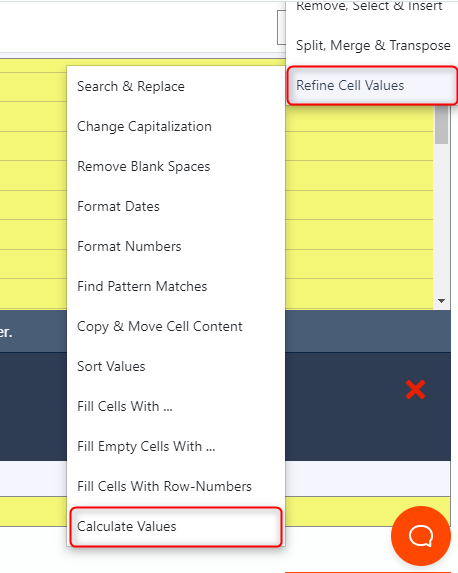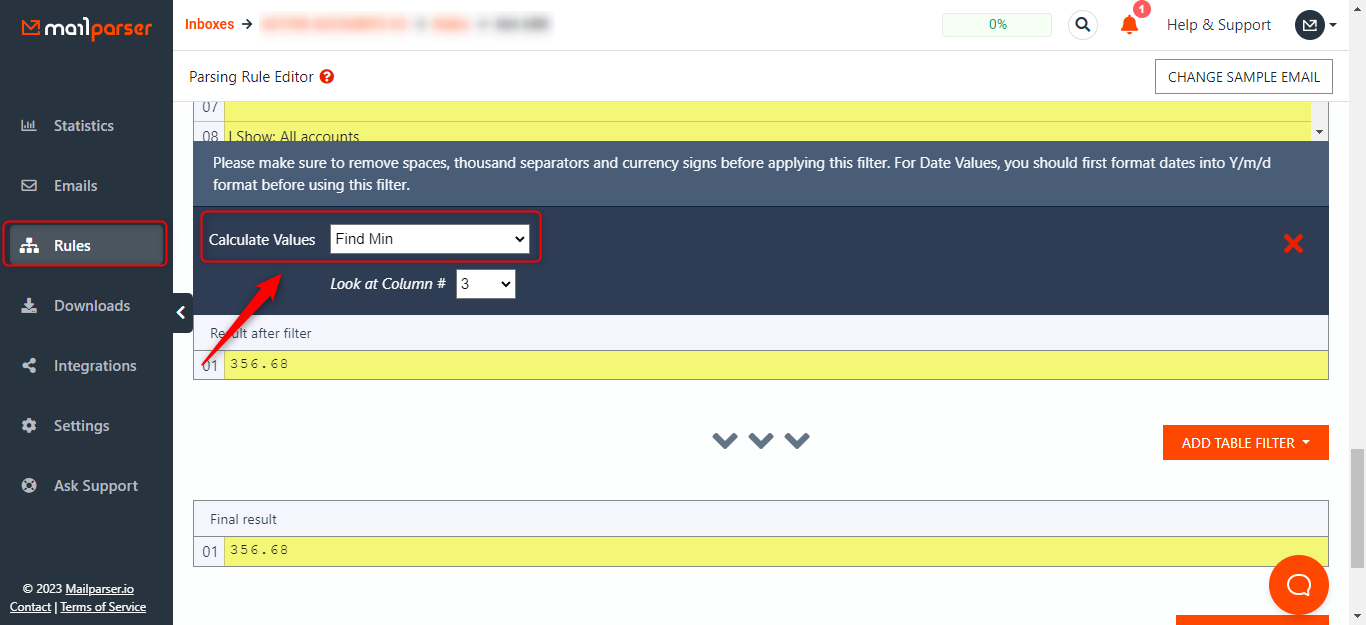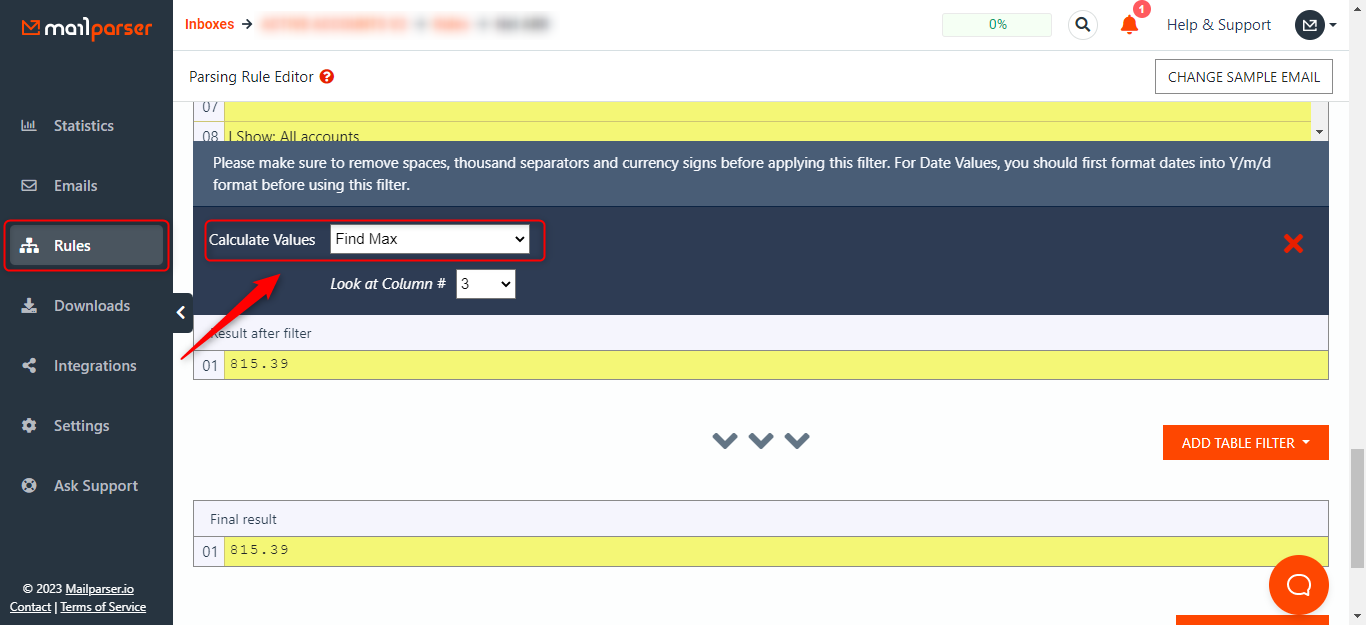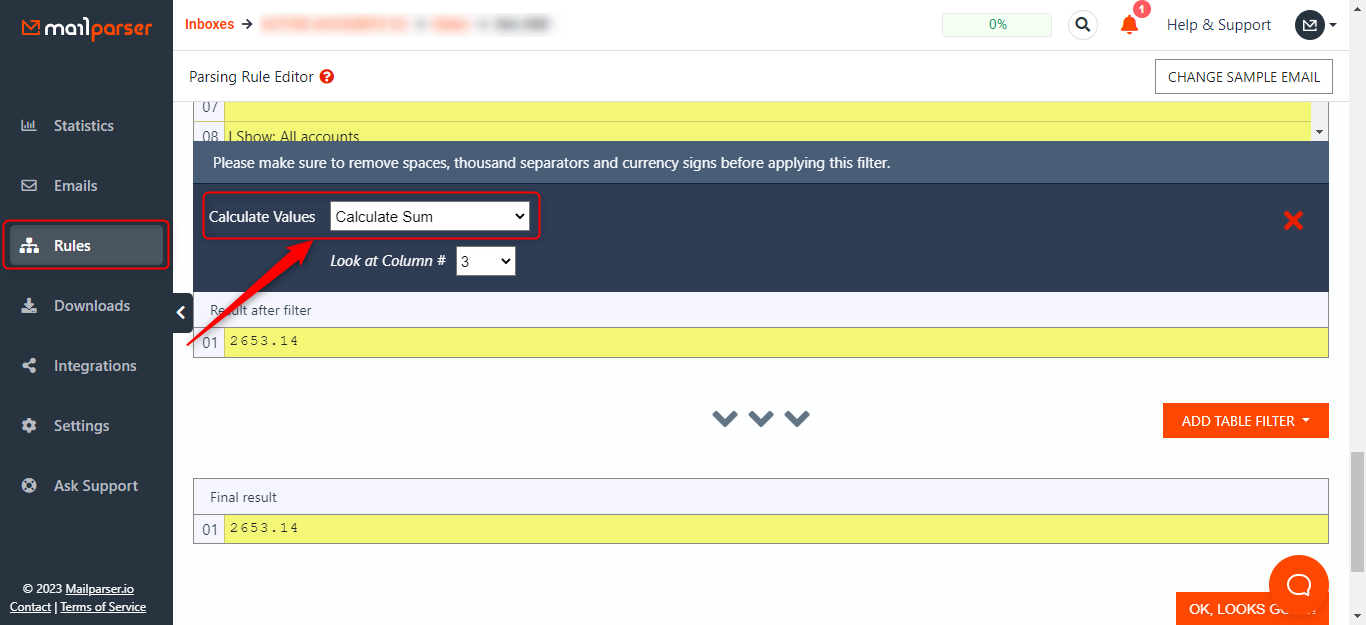# How do I use the Calculate Values filter?

If you need to calculate a minimum, maximum, or sum of values using the data in a column you can use this filter in the menu: Add table filter > Refine cell values > Calculate values filter:To calculate the minimum value of the column, select the column you want to calculate and then select Find Min.To calculate the maximum value of the column, select the column you want to calculate and then select Find Max.To calculate the all-sum value of the column, select the column you want to calculate and then select Calculate Sum.If you have any questions or run into trouble getting set up please reach out to us at support@mailparser.io.